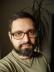## Product SubArray

Given a list of 4 or more numbers, write a script to find the contiguous sublist that has the maximum product. The length of the sublist is irrelevant; your job is to maximize the product.

Example

Input: `[ 2, 5, -1, 3 ]`

Output: `[ 2, 5 ]` which gives maximum product `10`.

The easiest (but probably not the fastest) method would be to start from each position and compute the products of all the possible sublists starting at the position, remembering the positions where the product is maximal.

I automatically reached for List::Util’s `product` to get the products easily, but alas! Running

``product(-1, 3)``

caused a Floating point exception (bug reported). So, I had to implement `product` myself:

## Excel Column

Write a script that accepts a number and returns the Excel Column Name it represents and vice-versa.

Excel columns start at A and increase lexicographically using the 26 letters of the English alphabet, A..Z. After Z, the columns pick up an extra “digit”, going from AA, AB, etc., which could (in theory) continue to an arbitrary number of digits. In practice, Excel sheets are limited to 16,384 columns.

Example

```Input Number: 28
Output: AB

Output: 30```

This seemed like a simple base 10 to base 26 conversion and back. I started by installing Math::Base::Convert, Math::BaseConvert, Math::BaseCnv, and Convert::AnyBase to quickly discover they wouldn’t help me much. What Excel uses for column names is a weird 26 digit system that lacks a symbol for zero, but has a symbol for 26 (or for 1026). It’s called the bijective base-26 numeration system. The interesting fact about such systems is that digit addition, subtraction, and multiplication work the same way as in our common system (division is a bit problematic).

## Perl Weekly Challenge 059: Linked List and Bit Sum

You are given a linked list and a value k. Write a script to partition the linked list such that all nodes less than k come before nodes greater than or equal to k. Make sure you preserve the original relative order of the nodes in each of the two partitions.

For example:

```Linked List: 1 → 4 → 3 → 2 → 5 → 2
k = 3
Expected Output: 1 → 2 → 2 → 4 → 3 → 5.```

We saw Linked List not so long ago, when solving the LRU Cache. Nevertheless, I didn’t reuse my solution, as I previously used a cyclic variant which doesn’t seem to be helpful here.

So, let’s start with a class of list elements. I call them “nodes”. Each node has a value and a link to a next node (undef if there’s none). A node can be disconnected from the next node, or a new node can be attached to it.

## Compare Version

Compare two given version number strings v1 and v2 such that:
• If v1 > v2 return 1
• If v1 < v2 return -1
• Otherwise, return 0

The version numbers are non-empty strings containing only digits, and the dot (“.”) and underscore (“_”) characters. (“_” denotes an alpha/development version, and has a lower precedence than a dot, “.”). Here are some examples:

v1v2Result
0.1<1.1-1
2.0>1.21
1.2<1.2_5-1
1.2.1>1.2_11
1.2.1=1.2.10

When I read the task assignment, I thought to myself: I’m not the first person in the world that needs to compare versions. There already must be a module on CPAN that does exactly that. As usually, it wasn’t so simple.

## Shortest Unique Prefix

Write a script to find the shortest unique prefix for each each word in the given list. The prefixes will not necessarily be of the same length.

Sample Input

`[ "alphabet", "book", "carpet", "cadmium", "cadeau", "alpine" ]`

Expected Output

`[ "alph", "b", "car", "cadm", "cade", "alpi" ]`I blog about Perl.